# Related Videos

## Solve the product power $\sqrt{24\cdot 6}$

Go!
1
2
3
4
5
6
7
8
9
0
a
b
c
d
f
g
m
n
u
v
w
x
y
z
.
(◻)
+
-
×
◻/◻
/
÷
2

e
π
ln
log
log
lim
d/dx
Dx
|◻|
=
>
<
>=
<=
sin
cos
tan
cot
sec
csc

asin
acos
atan
acot
asec
acsc

sinh
cosh
tanh
coth
sech
csch

asinh
acosh
atanh
acoth
asech
acsch

### Videos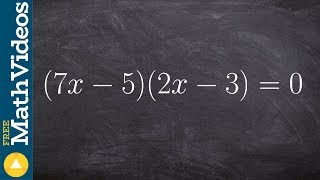### Algebra 2 - Using zero product property with two binomials multiplied by each other, (7x-5)(2x-3)=0

https://www.youtube.com/watch?v=hqOoC-p1LVE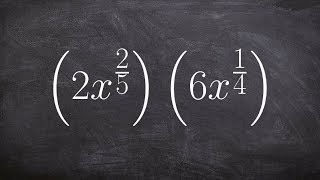### Algebra 2 - Multiplying two exponents with rational powers, then simplifying, 2x^(2/5) . 6x^(1/4)

https://www.youtube.com/watch?v=ITQM50h5BlY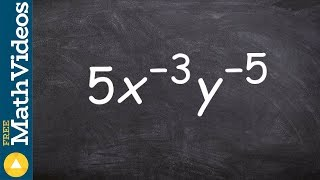### Tutorial - Rewriting exponents with positive powers to simplify an expression ex 19, 5x^-3 y^-5

https://www.youtube.com/watch?v=9J6rE1twTh4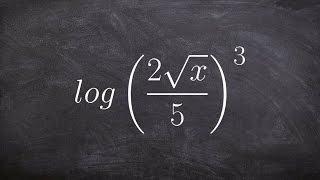### Tutorial - Expanding a logarithmic expression raised to the third power ex 17, log ((2root(x))/5)^3

https://www.youtube.com/watch?v=C74lT2ZterU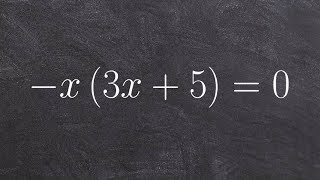### Algebra 2 - Solving a factored quadratic equation using the zero product property, -x(3x + 5) = 0

https://www.youtube.com/watch?v=3on6rsKoK_k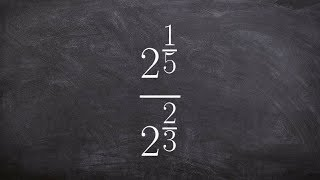### Tutorial - Learn how to divide two exponents with fractional powers, 2^(1/5) / 2^(2/3)

https://www.youtube.com/watch?v=MgPs8r_meRg
$\sqrt{24\cdot6}$

### Main topic:

Power of a product

~ 0.01 s